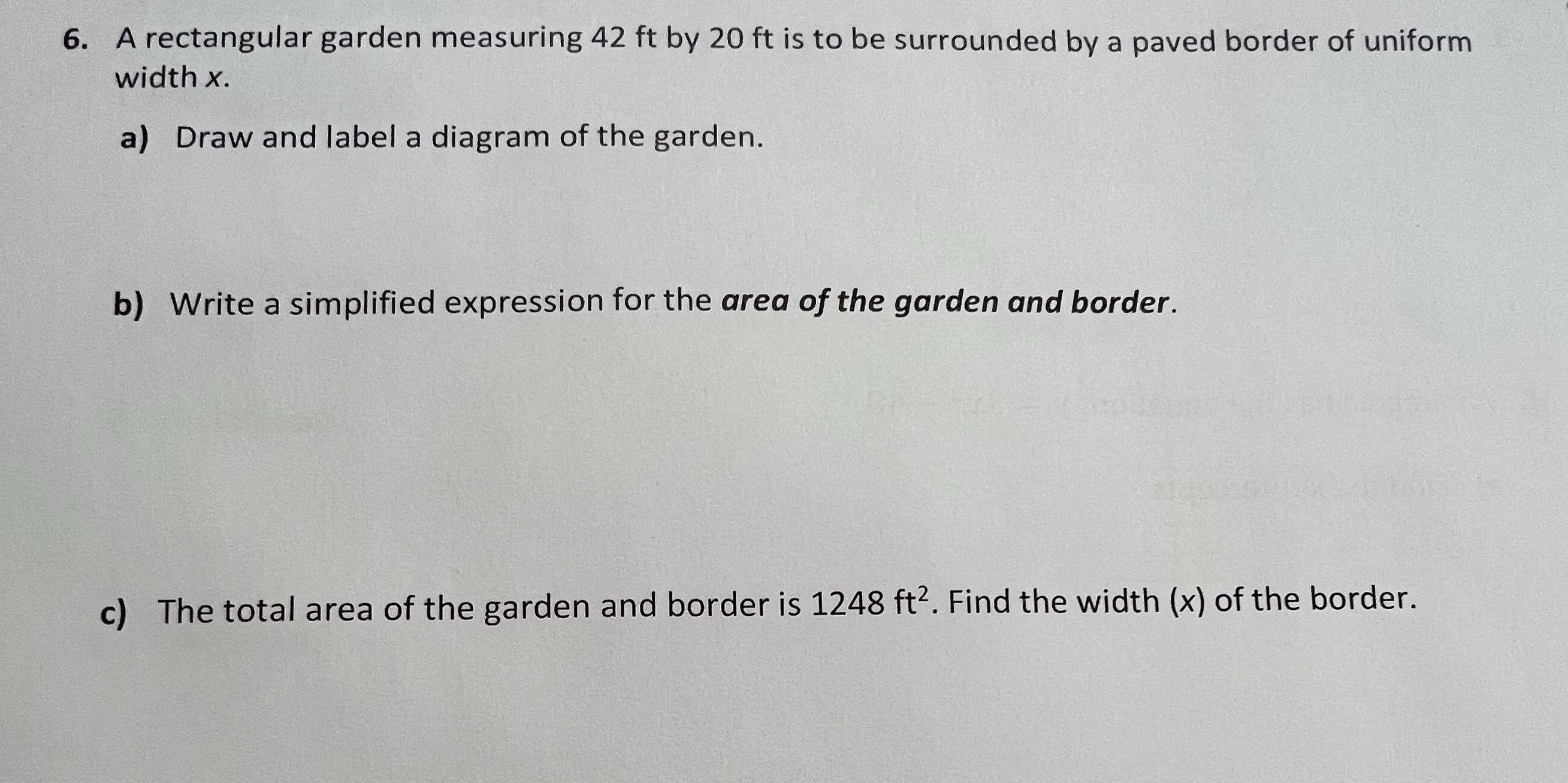### ¿Todavía tienes preguntas de matemáticas?

Pregunte a nuestros tutores expertos
Algebra
Pregunta6. A rectangular garden measuring $$42 ft$$ by $$20 ft$$ is to be surrounded by a paved border of uniform width $$x$$ . a) Draw and label a diagram of the garden. b) Write a simplified expression for the area of the garden and border. c) The total area of the garden and border is $$1248 ft ^ { 2 }$$ . Find the width $$( x )$$ of the border.

b) $$4x^{2} + 124x+ 840$$